Bivariate diagrams are used to display the relationship between two sets of variables. Each axis can be a single variable or be the sum of multiple variables.

One popular type of bivariate diagram is a TAS plot. TAS is an acronym for Total Alkali vs. Silica. This type of plot is used to classify igneous rocks by comparing the weigh percentage of SiO2 with the weight percentage of NaO2 plus K20 in a particular rock sample. Specific ranges of this ratio are assigned a specific compositional classification.

Below is a classic example of an extrusive TAS bivariate plot, modelled after the 1986 puplication by Le Bas, et al.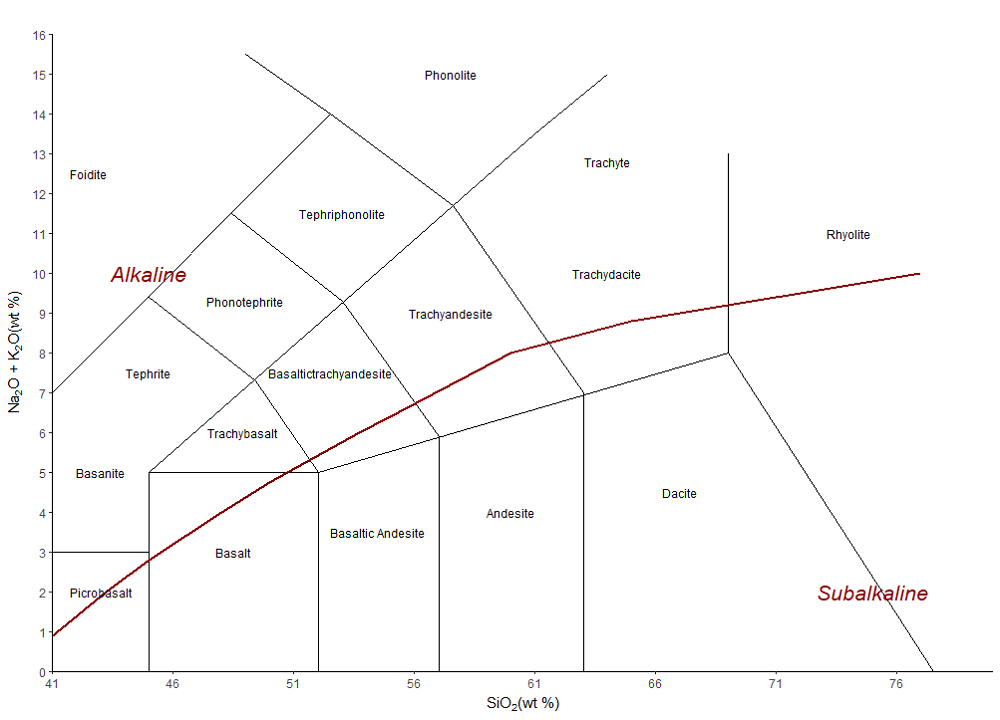#### Control geometries for each group below#### Select Discrimination Plot:

Ternary diagrams are triangular plots of three variables that sum to a constant, typically 100%. First introduced by August Mobius in 1827, they are sometimes called simplex plots, Gibbs triangles, or de Finetti diagrams. Ternary diagrams are commonly used within the geosciences as classification/discrimination diagrams.

Below are examples of common ternary diagrams used in the earth sciences: the USDA soils classification diagram and the Sheperd’s Sediment classification diagram.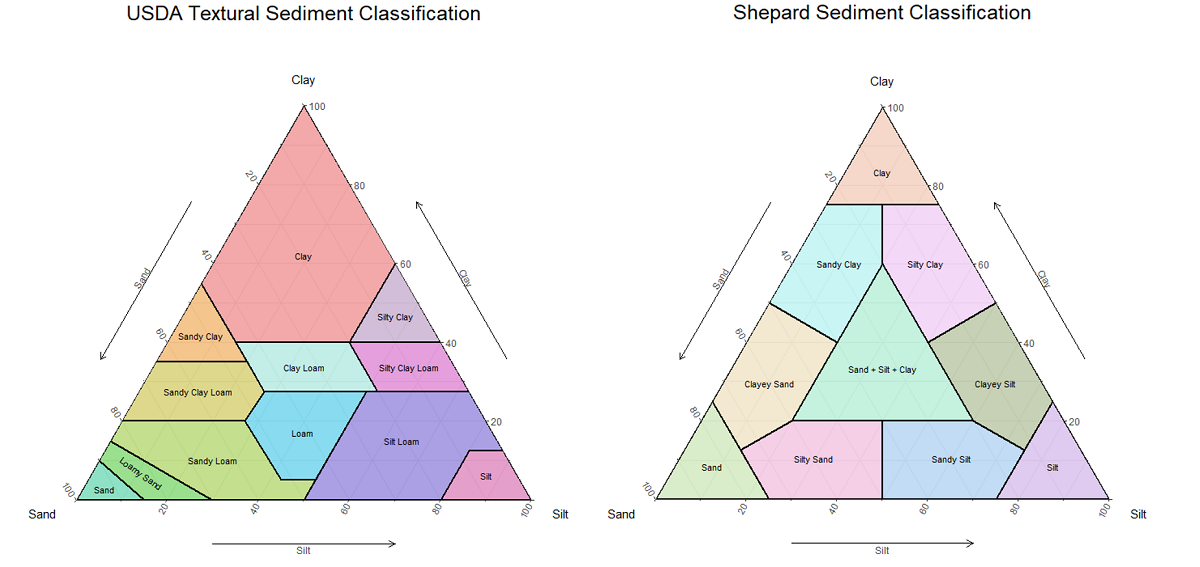Other notable ternary diagrams in the earth sciences include the QFL diagram, QAPF diagram, and a large variety of ternary phase diagrams.

## Plotting Onto a Ternary Diagram

Ternary diagrams make the assumption that the three variables plotted make up 100% of the sample. Before you can plot a data point (say, A = 700, B = 200, and C = 100) you must first normalize that data to 100%. This is done by dividing each value for a point (A, B, and C) by the sum of those values. The generalized equation for this is below: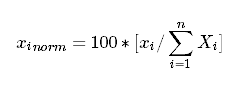For value A in our example above this becomes: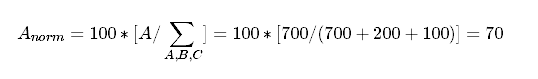Thus, after normalization the above data point becomes (A = 70, B = 20, C = 10).

Adding a point to a ternary diagram is relatively simple once you get your head around how points move along the axes. Because we plot normalized data onto a ternary plot, all axes are in units of percent (%) and the values range from 0 to 100 (sometimes 0 to 1). The maximum for each ternary axis is located at the triangle node with the axis label. Zero for the any ternary axis is located along the line opposite the axis label. So, to move along a ternary axis you start at the line opposite the axis label and then move perpendicularly away from the line toward the axis label.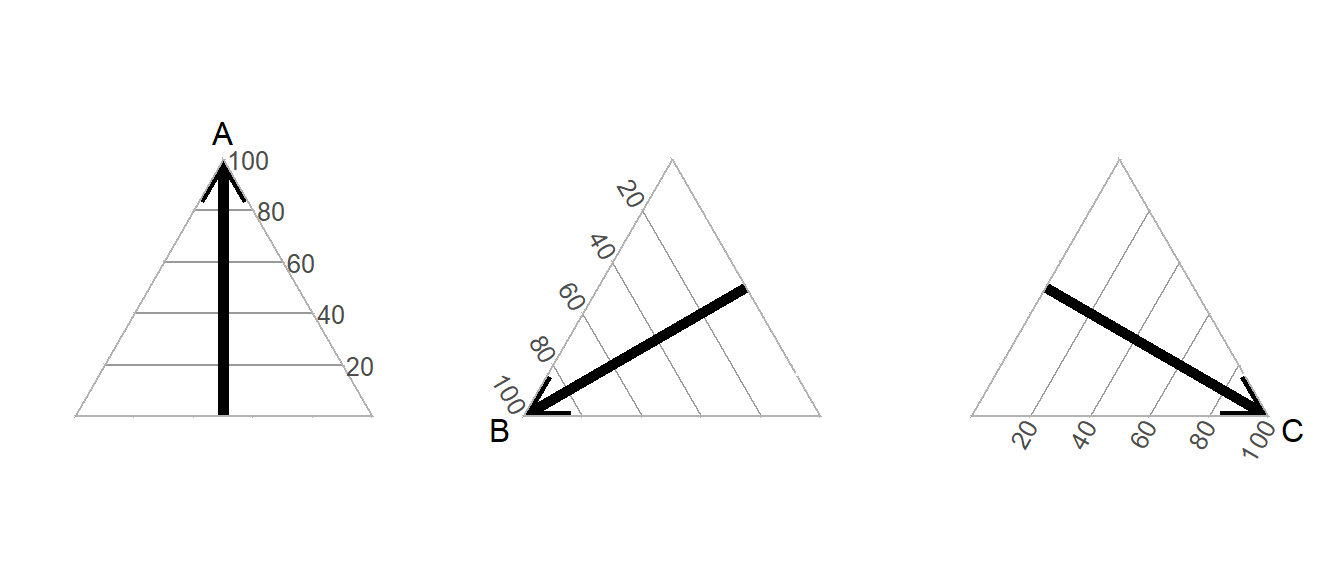Thus, our example point from above (A = 70, B = 20, C = 10) will plot on a ternary diagram like so.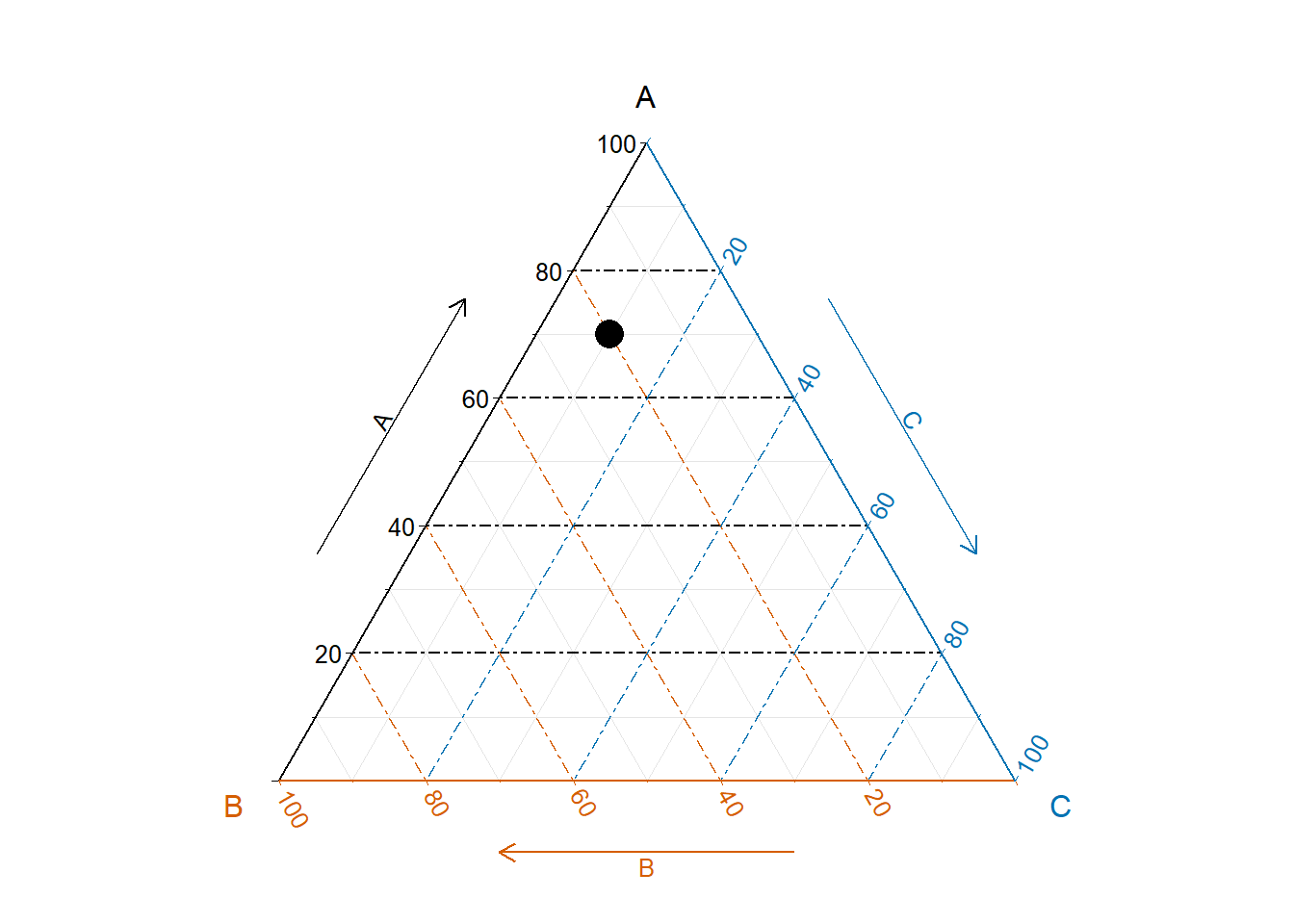In practice, you will generally only need two coordinates to find a point location on a ternary plot. This is because ternary points always sum to 100, so after plotting a point on axis A and B, axis C will be solved.

## Pay attention to the axes!

It is important to note the axes of a ternary plot can rotate clockwise or counter-clockwise without changing the position where a point will plot. Only the order of the axes around the triangle controls where a point will plot. For example, the point below does not shift if the axis rotation changes; however, if the arrangement of axes changes, it shifts.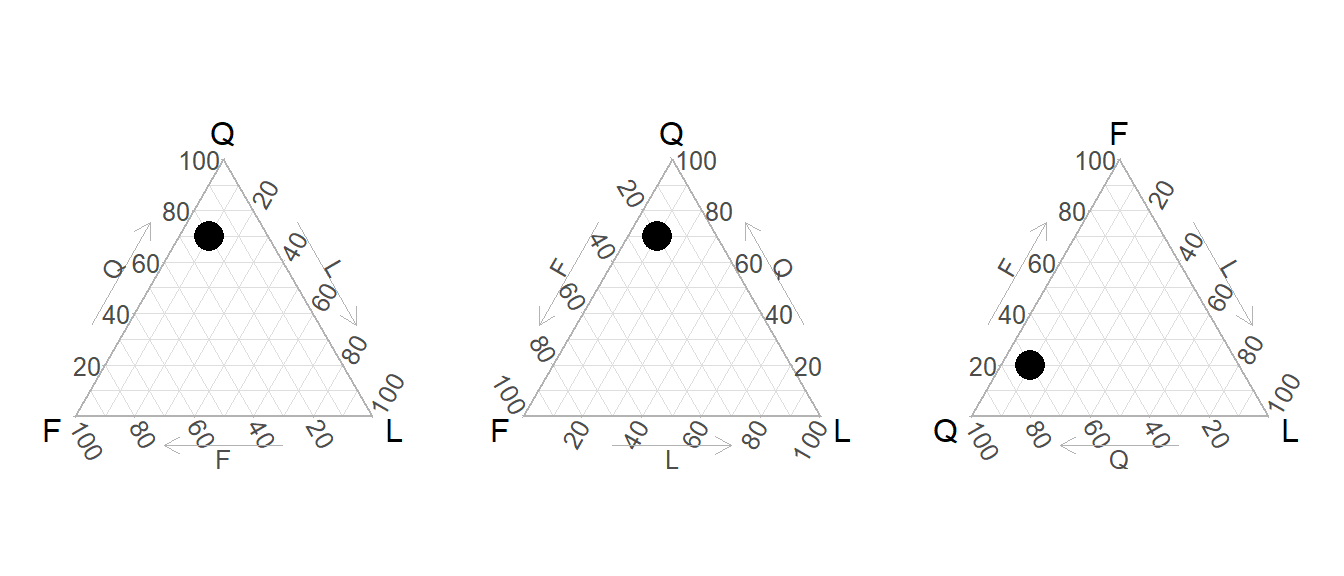### Interactive Ternary Diagram

#### Control geometries for each group below#### Select Discrimination Plot:

A metamorphic pressure vs. temperature plot shows the locations of metamorphic facies within pressure-temperature space. Individual facies are defined by specific mineralogical assemblages, which are consistent throughout a given facies.

Metamorphic facies were first introduced by Finnish geologist Pentti Eskola in 1920. Click here to read his original manuscript. These were later expanded on by New Zealand geologist and UC Berkeley professor Francis John Turner.

Below is a typical metamorphic facies diagram.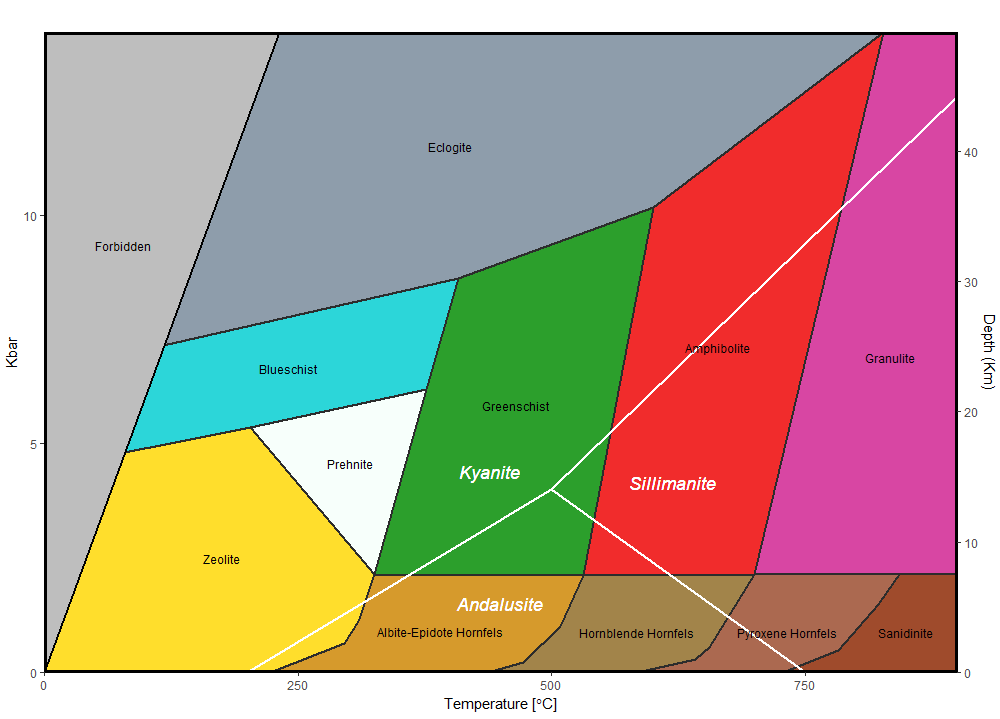#### Control geometries for each group below## Open Access Publications

These individual publication links are all available via the Mineral Society of America Open Access Publications List.

# How to Load Data into the RockR! App: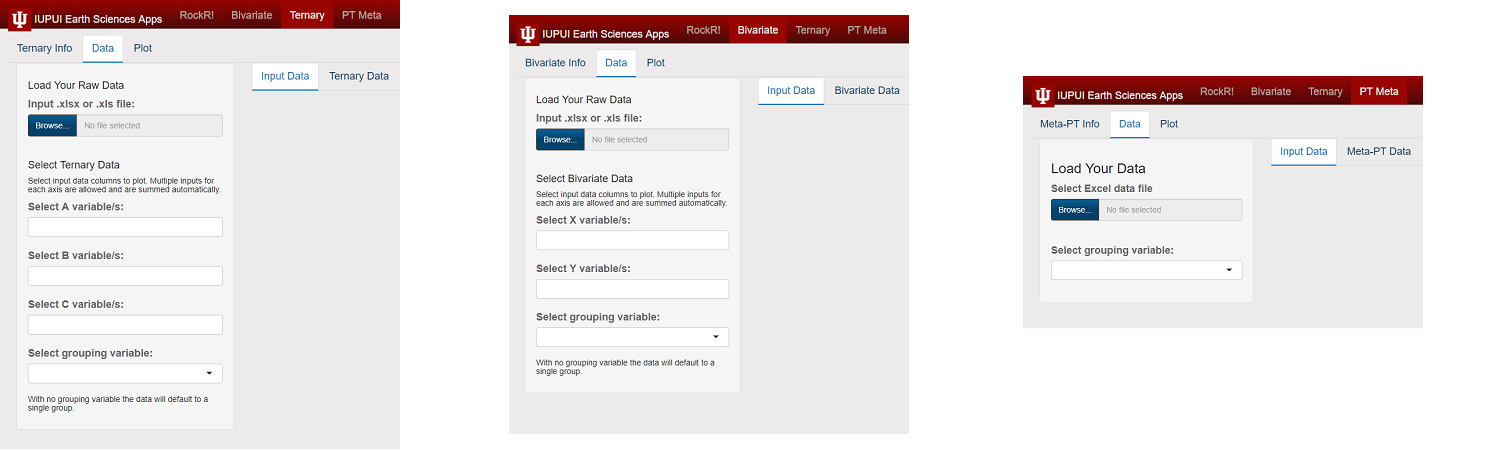Click on the browse button to select a .csv, .xlsx, or .xls file to load. The “Input Data”" tab and the plot-specific data tab on the right will remain blank until a dataset has been loaded.

After loading, the “Input Data” tab will populate with the entire data file, though only the first 10 entries (rows) will display by default. This can be adjusted to 25, 50, or 100 entries per page in the drop-down menu under these tabs.

Select the columns to be represented by each variable and then an optional grouping variable can be chosen. The grouping variable can be any column in the data set, and helps organize the data by location, date, or whatever characteristic applies to the data set as a whole. Once the variables have been selected, with or without the optional grouping variable, the plot-specific tab (either Ternary Data, Bivariate Data, or Metamorphic Facies data) will display only the selected data relevant to the plot. For the Bivariate and Metamorphic Facies tabs, this will include the X variable, the Y variable, and the grouping variable (if one was selected). For the Ternary tab, this will include the A variable, the B variable, the C variable, and the grouping variable (if one was selected).

If multiple selections were made for a given variable, this will be shown as an aggregate value.

# Good practices for data organization

Values in the spreadsheet should be only numerical with the exception of column titles and values in a column used for grouping.

Refrain from including hyphens in text values because R interprets a “-” symbol as subtraction instead of a hyphen. This also applies to other common numerical operators.

In a situation where a group variable includes multiple words, separate the words by underscores instead of spaces (i.e. Use “Sample_group” instead of “Sample group”).

# Understanding tidy data

RockR! loads data as .xls, .xlsx, or .csv files. Additionally, RockR! expects data to be tidy in its form. Tidy data has rows that represent samples and columns that represent variables.